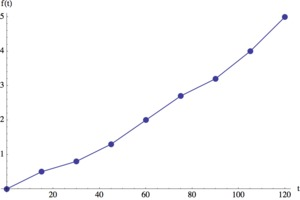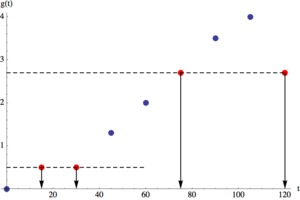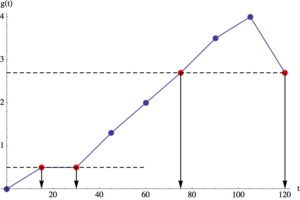# Invertible or Not?

Alignments to Content Standards: F-BF.B.4

The table below shows some input-output pairs of two functions $f$ and $g$ that agree for the values that are given but some of their output values are missing.

 $t$ $f(t)$ $g(t)$ 0 15 30 45 60 75 90 105 120 0 0.5 1.3 2 2.7 4 0 0.5 1.3 2 2.7 4
1. Complete the table in a way so that $f$ could be invertible and so that $g$ is definitely not invertible.
2. Graph both functions and explain from the graph why $f$ is invertible and $g$ is not.
3. Come up with two real life situations that $f$ and $g$ could be representing.
4. Find and interpret the value $f^{-1}(4)$ in terms of these contexts.

## IM Commentary

This task illustrates several components of standard F-BF.B.4.c: Find Inverse Functions. Here, instead of presenting two functions and asking the students to decide which one is invertible, students are asked to complete a table of input-output pairs for the functions in such a way that one of the functions is invertible and the other one is not.

In part (c) students are asked to envision contexts plausibly represented in the table, and then in part (d), students are asked to find and interpret the "inverse context." This task also directly addresses part (c) of F-BF.4, which asks students to read values of inverse functions from a table. Finally, the task further gives students an opportunity to contrast an invertible and non-invertible function, which is mentioned in the Functions Progressions document as a reasonable extension of the standard. "...And although not required in the standard, it is reasonable to include, for comparison, a few examples where the input cannot be uniquely determined from the output." (pg. 13 Functions Progression)

The task could be used for instruction or assessment.

## Solution

1. There are two natural ways to interpret the domain of the given functions. Either, we assume that the table shows the entire domain, so the domain consists of discrete points, or we assume that the domain of the given functions are all real numbers between 0 and 120. With the former interpretation, to complete the table for $f$, we just have to make sure that we don't repeat output values. With the latter interpretation, we have to choose output values that make the function strictly increasing from $t=0$ to $t=120$. To make sure that $g$ is not invertible, we can repeat output values. The values given in the table below work for both interpretations of the domain. (There are many more domains we could work with, but we will just show solutions for theses two.)

 $t$ $f(t)$ $g(t)$ 0 15 30 45 60 75 90 105 120 0 0.5 0.8 1.3 2 2.7 3.2 4 5 0 0.5 0.5 1.3 2 2.7 3.5 4 2.7
2. We show graphs of both cases, discrete domain and continuous domain.We can see that every output value of $f$ can be traced back to a unique input value. Therefore, $f$ is invertible.We can see that there are some output values that can be traced back to several input values, for example $g(t)=0.5$ and $g(t)=2.7$. Therefore, $g$ is not invertible.

3. There are many possible answers. For example, $f(t)$ could be the height of a plant, in inches, after $t$ days. If the plant continues to grow, then it makes sense that every output can be traced back to a unique input and so the function $f$ is invertible.

We could have $g(t)$ be the depth of the snow on the ground, in inches, after $t$ hours. Looking at the way we completed the table, it started to snow but then stopped and there were 0.5 inches on the ground for a while. Then it continued to snow and the depth of the snow increased. At the end of the time shown in the table, the snow depth actually decreased. So if we wanted to know at what time there were 2.7 inches of snow on the ground, we would not be able to give a unique answer. The snow depth was 2.7 inches twice, after 75 and 120 hours.

4. To find $f^{-1}(4)$ we have to trace back the output $4$ of function $f$ to the corresponding input. In other words, we have to find $t$ such that $f(t)=4$. In the above plant context, we are looking for the time when the plant was 4 inches high. We learn from the table that this happened after $t=105$ days.